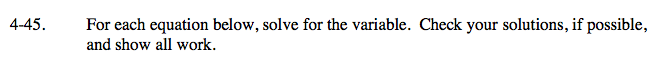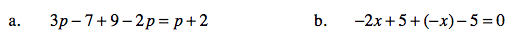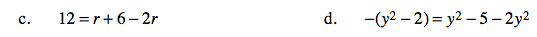### Home > CAAC > Chapter 4 > Lesson 4.1.5 > Problem4-45

4-45.For more help with parts (a) - (d), see the equation mat in problem 2-105.First, try rewriting all of the subtraction as addition, then attempt to solve by simplifying like terms.

3p + (−7) + 9 + (−2p) = p + 2
p + 2 = p + 2
p = p

Since p = p (or 2 = 2, depending on how you solved the problem),
p can equal any number.One strategy is to rewrite all of the subtraction within the problem as addition.
y2 + 2 = y2 + − 5 + −2y2

Now simplify, starting with combining like terms and then with isolating the variable.

y2 + 2 = −y2 + −5
2 = −5

No solution because 2 is not equal to −5.